# 13. Data Structures for Integers

In this chapter, we return to the problem of implementing an SSet. The difference now is that we assume the elements stored in the SSet are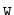-bit integers. That is, we want to implement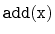,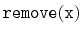, and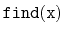where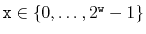. It is not too hard to think of plenty of applications where the data--or at least the key that we use for sorting the data--is an integer.

We will discuss three data structures, each building on the ideas of the previous. The first structure, the BinaryTrie performs all three SSet operations in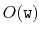time. This is not very impressive, since any subset ofhas size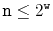, so that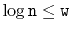. All the other SSet implementations discussed in this book perform all operations in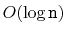time so they are all at least as fast as a BinaryTrie.

The second structure, the XFastTrie, speeds up the search in a BinaryTrie by using hashing. With this speedup, theoperation runs in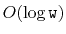time. However,andoperations in an XFastTrie still taketime and the space used by an XFastTrie is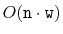.

The third data structure, the YFastTrie, uses an XFastTrie to store only a sample of roughly one out of everyelements and stores the remaining elements a standard SSet structure. This trick reduces the running time ofandtoand decreases the space to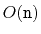.

The implementations used as examples in this chapter can store any type of data, as long an integer can be associated with it. In the code samples, the variable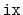is always the integer value associated with, and the method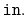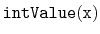convertsto its associated integer. In the text, however, we will simply treatas if it is an integer.

Subsections
opendatastructures.org